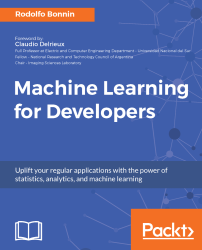•#### Machine Learning for Developers#### Overview of this book

Most of us have heard about the term Machine Learning, but surprisingly the question frequently asked by developers across the globe is, “How do I get started in Machine Learning?”. One reason could be attributed to the vastness of the subject area because people often get overwhelmed by the abstractness of ML and terms such as regression, supervised learning, probability density function, and so on. This book is a systematic guide teaching you how to implement various Machine Learning techniques and their day-to-day application and development. You will start with the very basics of data and mathematical models in easy-to-follow language that you are familiar with; you will feel at home while implementing the examples. The book will introduce you to various libraries and frameworks used in the world of Machine Learning, and then, without wasting any time, you will get to the point and implement Regression, Clustering, classification, Neural networks, and more with fun examples. As you get to grips with the techniques, you’ll learn to implement those concepts to solve real-world scenarios for ML applications such as image analysis, Natural Language processing, and anomaly detections of time series data. By the end of the book, you will have learned various ML techniques to develop more efficient and intelligent applications.
PrefaceFree Chapter
Introduction - Machine Learning and Statistical ScienceThe Learning ProcessClusteringLinear and Logistic RegressionNeural NetworksConvolutional Neural NetworksRecurrent Neural NetworksRecent Models and DevelopmentsSoftware Installation and Configuration# The Learning Process

In the first chapter, we saw a general overview of the mathematical concepts, history, and areas of the field of machine learning.

As this book intends to provide a practical but formally correct way of learning, now it's time to explore the general thought process for any machine learning process. These concepts will be pervasive throughout the chapters and will help us to define a common framework of the best practices of the field.

The topics we will cover in this chapter are as follows:

• Understanding the problem and definitions
• Dataset retrieval, preprocessing, and feature engineering
• Model definition, training, and evaluation
• Understanding results and metrics

Every machine learning problem tends to have its own particularities. Nevertheless, as the discipline advances through time, there are emerging patterns of what kind of steps a machine learning...